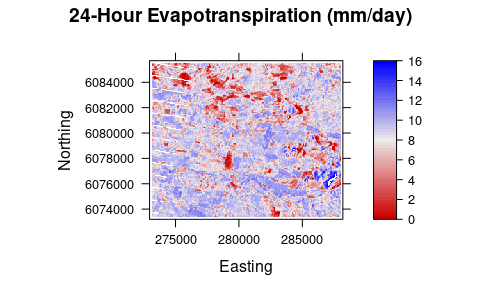Surface Energy Balance using METRIC model and water package: 2. advanced procedure

2018-04-24

This vignette presents the procedure to estimate the Land Surface Energy Balance (LSEB) using lansat imagery and The water package. There are two version of this vignette: simple and advanced version. In the first one the procedure is simpler but most of the parameters are selected automatically by the package. In the second aproach, the procedure is longer because the user has more control on all input parameters and coefficent. Both vignettes follow the METRIC model methodology (Allen et la., 2007) in order to estimate the LSEB using landasat 7 and 8 satellite images.

Also, on the simple procedure we are going to assume flat terrain, but in the advanced procedure we are going to use a digital elevation model (DEM). However, is possible to use a digital elevation model in the simple procedure, changing the parameter flat to FALSE and providing a DEM.

Introduction

One of the most cited models to estimate land surface evapotranspiration from satellite-based energy balance is the Mapping EvapoTranspiration at high Resolution with Internalized Calibration (METRIC). This model was developed by Allen et al., (2007) based on the well-known SEBAL model (Bastiaanssen, 1998). It has been widely applied in many countries arround the world to estimate crops evapotranspiration (ET) at field scales and over large areas using satellite images. The model it has been apply in different vegetation and crops types such us, wheat, corn, soybean and alfalfa with good results (3 - 20% of error) and also in recent years over sparce woody canopies such us vineyards, and olive orchards, in both plain and mountainous terrain. Thus, ET is estimated as a residual of the surface energy equation: $\begin{equation} \label{eq:EB} LE = R_n - G - H \end{equation}$ where $$LE$$ is latent energy consumed by ET ($$W \cdot m^{-2}$$); $$Rn$$ is net radiation ($$W \cdot m^{-2}$$); $$G$$ is sensible heat flux conducted into the ground ($$W \cdot m^{-2}$$); and $$H$$ = sensible heat flux convected to the air ($$W \cdot m^{-2}$$).

We estimate $$Rn$$, $$G$$ and $$H$$ for each pixel into a Landsat satellite scene, supported by one weather station. Then we estimate $$LE$$ fluxes using previous equation, and after that, the instantaneous evapotranspiration values as:

$\begin{equation} ET_{inst} = 3600 \cdot \frac{LE}{\lambda \rho_w} \end{equation}$

where $$ET_{inst}$$ is the instantaneous ET at the satellite flyby ($$mm \cdot h^{-1}$$); 3600 is the convert factor from seconds to hours; $$\rho_w$$ is density of water = 1000 $$kg\cdot m^{-3}$$; and $$\lambda$$ is the water latent heat of vaporization ($$J\cdot kg^{-1}$$).

Finally the daily ET is computed pixel by pixel (30 x 30 m) as: $\begin{equation} ET_{24} = \frac{ET_{inst}}{ET_r} ET_{r\_24} \label{eq:et24} \end{equation}$

To begin this procedure, first we have to load water package:

library(water)

Base data preparation

To calculate METRIC crops Evapotranspiration using water package and the simple procedure, we’re going to use three sources:

• A raw Landsat 7/8 satellite image (original .TIF data from glovis USGS).
• A Weather Station data (.CSV file).
• A polygon with our Area-of-interest (AOI) Spatial-Polygon object (if we wont estimate corp ET for the entire landsat scene).

First, we create the AOI as a polygon using bottomright and topleft points:

aoi <- createAoi(topleft = c(272955, 6085705),
bottomright = c( 288195, 6073195), EPSG = 32719)

Then, we load the weather station data. For that we are going to use the function called read.WSdata. This function converts our .csv file into a waterWeatherStation object. Then, if we provide a Landsat metadata file (.MTL file) we will be able to calculate the time-specific weather conditions at the time of satellite overpass

csvfile <- system.file("extdata", "apples.csv", package="water")
MTLfile <- system.file("extdata", "L7.MTL.txt", package="water")
WeatherStation <- read.WSdata(WSdata = csvfile, date.format = "%d/%m/%Y",
lat=-35.42222, long= -71.38639, elev=201, height= 2.2,
columns=c("date" = 1, "time" = 2, "radiation" = 3,
"wind" = 4, "RH" = 6, "temp" = 7, "rain" = 8),
MTL = MTLfile)
## Warning in read.WSdata(WSdata = csvfile, date.format = "%d/%m/%Y", lat =
## -35.42222, : As tz = "", assuming the weather station time zone is America/
## Argentina/Buenos_Aires

We can visualize our weather station data as:

print(WeatherStation, hourly=FALSE)
## Weather Station @ lat: -35.42 long: -71.39 elev: 201
## Summary:
##  Min.   :  0.00   Min.   : 0.000   Min.   :17.39   Min.   :0.7455
##  1st Qu.:  0.00   1st Qu.: 0.205   1st Qu.:40.65   1st Qu.:1.2288
##  Median : 49.82   Median : 1.585   Median :64.83   Median :1.6472
##  Mean   :310.13   Mean   : 3.071   Mean   :60.78   Mean   :1.5156
##  3rd Qu.:719.29   3rd Qu.: 3.655   3rd Qu.:82.34   3rd Qu.:1.7741
##  Max.   :998.29   Max.   :14.460   Max.   :94.04   Max.   :1.9672
##       temp            rain
##  Min.   :14.65   Min.   :0
##  1st Qu.:17.74   1st Qu.:0
##  Median :21.15   Median :0
##  Mean   :22.46   Mean   :0
##  3rd Qu.:27.36   3rd Qu.:0
##  Max.   :32.53   Max.   :0
##
##  Conditions at satellite flyby:
##               datetime radiation wind    RH   ea  temp rain       date
## 47 2013-02-15 11:30:40    752.92  1.1 68.86 1.89 22.59    0 2013-02-15
plot(WeatherStation, hourly=TRUE)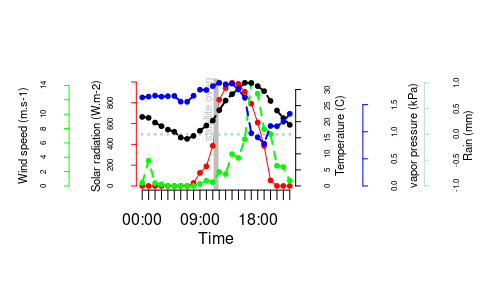After that, we load the Landast satellite image. Usually, using water we can use the function called loadImage to load a Landsat image from .TIF files were downloaded directly from Earth Explorer. In this vignette we are going to use some Landsat 7 as example data which, by the way comes with water package as a demo.

image.DN <- L7_Talca

Finally we are going to create The Digital Elevation Model (DEM) for the specific satellite image that we are processing. Thus, we needed a image-specifc grid files downloadable from Earth Explorer. Therefore, is needed to check wich grid files we are going to use considering the satellite scene location and the AOI polygon, using:

checkSRTMgrids(image.DN)
##  "You need 1 1deg x 1deg SRTM grids"
##  "You can get them here:"
##  "http://earthexplorer.usgs.gov/download/options/8360/SRTM1S36W072V3/"

You should download all needed grid files, and then you can use the function prepareSRTMdata(extent = image.DN) to create our DEM. In this vignette we are going to load the example data provided with water package.

DEM <- DEM_Talca

In order to calculate the Net Radiation from loaded landsat satellite data, first we calculate a surface model (slope + aspect) from the DEM, then we calulate the solar angles (latitude, declination, hour angle and solar incidence angle), and finally we plot the last one. Then we use this function to calculate incoming solar radiation.

surface.model <-METRICtopo(DEM)

solar.angles.r <- solarAngles(surface.model = surface.model,
WeatherStation = WeatherStation, MTL = MTLfile)

plot(solar.angles.r)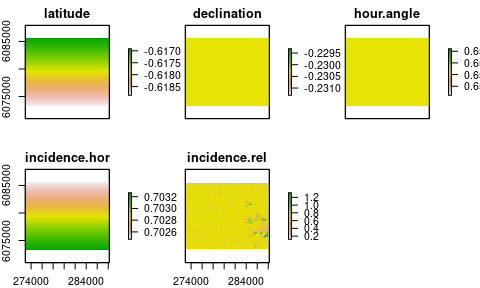Rs.inc <- incSWradiation(surface.model = surface.model,
solar.angles = solar.angles.r,
WeatherStation = WeatherStation)

Then we calculate reflectances at the top-of-atmosphere (TOA), and surface reflectance derived from Landsat image, and use the last one to calculate the broadband albedo as:

image.TOAr <- calcTOAr(image.DN = image.DN, sat="L7", MTL = MTLfile,
incidence.rel = solar.angles.r$incidence.rel) image.SR <- calcSR(image.TOAr=image.TOAr, sat = "L7", surface.model=surface.model, incidence.hor = solar.angles.r$incidence.hor,
WeatherStation=WeatherStation)

albedo <- albedo(image.SR = image.SR,  coeff="Tasumi", sat="L7")

Later on, we calculate the Leaf Area Index (LAI) using the satellite data, and we plot it. In this step, we can choose diferents methods able in literature to estimate LAI from Landsat data (see package Help for more info.). In this vignette we are going to use the METRIC 2010 method:

LAI <- LAI(method = "metric2010", image = image.TOAr, L=0.1)

plot(LAI)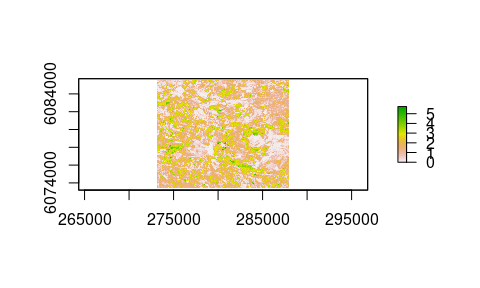Then we estimate land surface temperature (Ts), using computed LAI in order to estimate consequently the surface emissivity, and brightness temperature from landsats thermal band (TIR). Then we use this information to compute the incoming and outgoing long-wave radiation as:

Ts <- surfaceTemperature(image.DN=image.DN, LAI=LAI, sat = "L7",
WeatherStation = WeatherStation)

Rl.out <- outLWradiation(LAI = LAI, Ts=Ts)

Rl.inc <- incLWradiation(WeatherStation,DEM = surface.model$DEM, solar.angles = solar.angles.r, Ts= Ts) And finally, we can estimate Net Radiation (R_n) pixel by pixel as follows: Rn <- netRadiation(LAI, albedo, Rs.inc, Rl.inc, Rl.out) plot(Rn)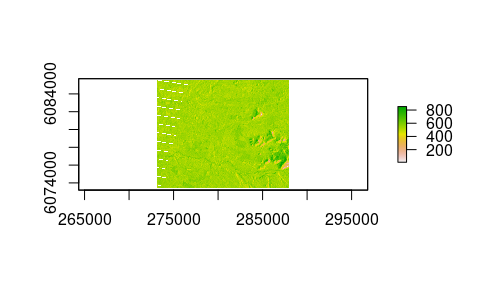Soil Heat Flux (G) estimation We estimate the soil heat flux using as an input data the Net radiation, surface reflectance, surface temperature, leaf area index and albedo. In this vignette we are going to use the original METRIC (2007) baded-method, which is: G <- soilHeatFlux(image = image.SR, Ts=Ts,albedo=albedo, Rn=Rn, LAI=LAI) plot(G)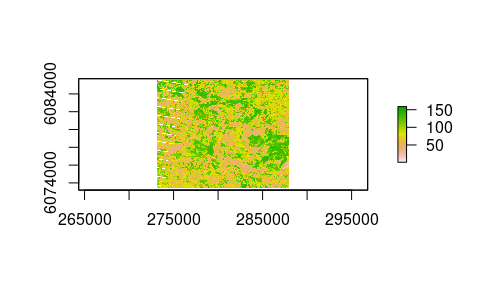Sensible Heat Flux (H) estimation To estimate the sensible heat fluxes derived from the landsat satellite data, first we need to calculate the Momentum roughness length (Zom). In this step, we are able to choose diferents methods found in literature to estimate Zom from Landsat data (see package Help for more information). In this vignette we are going to use the short.crops method. Then, we are going to use calAnchors function for automatically search end members within the satellite scene (extreme wet and dry conditions). In this vignette we are going to use the CITRA-MCB method (see Help for mor information). Finally we estimate the H values at pixel scales using calcH function. This function aplies the CIMEC self-calibration method in order generete an iteration process for the “hot” and “cald” pixels and to abdorb all baises in the computation of H. This iteration procces it will be able to see as a dinamic-plot when the function calcH is running. There is a parameter called verbose to control how much information we want to see in the output about the “anchor pixels” and iteration process. Z.om <- momentumRoughnessLength(LAI=LAI, mountainous = TRUE, method = "short.crops", surface.model = surface.model) hot.and.cold <- calcAnchors(image = image.TOAr, Ts = Ts, LAI = LAI, plots = F, albedo = albedo, Z.om = Z.om, n = 5, anchors.method = "CITRA-MCB", deltaTemp = 5, WeatherStation = WeatherStation, verbose = FALSE) ## Warning in calcAnchors(image = image.TOAr, Ts = Ts, LAI = LAI, plots = F, : anchor method names has changed. Old names (CITRA-MCBx) are ## deprecated. Options now include 'best', 'random' and 'flexible' ## Warning in calcAnchors(image = image.TOAr, Ts = Ts, LAI = LAI, plots = F, : ## I can only find 1 anchors with cold pixel conditions ## Warning in calcAnchors(image = image.TOAr, Ts = Ts, LAI = LAI, plots = F, : ## I can only find 1 anchors with hot pixel conditions H <- calcH(anchors = hot.and.cold, Ts = Ts, Z.om = Z.om, WeatherStation = WeatherStation, ETp.coef = 1.05, Z.om.ws = 0.03, DEM = DEM, Rn = Rn, G = G, verbose = FALSE)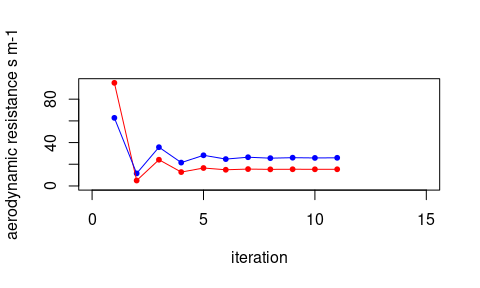Daily Crop Evapotranspiration (ET_24) estimation To estimate the daily crop evapotranspiration from the Landsat scene we need the daily reference ET (ETr) for our weather station, so we can calculate the daily ETr with: ET_WS <- dailyET(WeatherStation = WeatherStation, MTL = MTLfile) And finally, we can estimate daily crop ET pixel by pixel: ET.24 <- ET24h(Rn, G, H$H, Ts, WeatherStation = WeatherStation, ETr.daily=ET_WS)